### IMO Shortlist 1985 problem 17

Kvaliteta:
Avg: 0.0
Težina:
Avg: 0.0
The sequence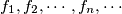$f_1, f_2, \cdots, f_n, \cdots$ of functions is defined for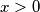$x > 0$ recursively by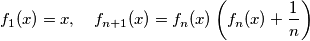Prove that there exists one and only one positive number$a$ such that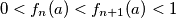$0 < f_n(a) < f_{n+1}(a) < 1$ for all integers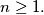$n \geq 1.$
Source: Međunarodna matematička olimpijada, shortlist 1985